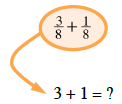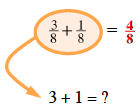Home > CC1MN > Chapter cc21 > Lesson cc21.2.5 > Problem1-106

1-106.

Rewrite each expression as a single fraction. Homework Help ✎

1. $\frac{3}{8}+\frac{1}{8}$

When adding or subtracting fractions with a common denominator, add or subtract with the numerators only.Reduce if possible.

1. $\frac{4}{5}+\frac{3}{4}$

See part (a).

1. $\frac{6}{7}-\frac{2}{5}$

See part (a).